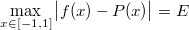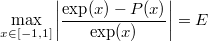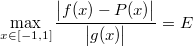# Remez tutorial 2/5: switching to relative error

In the previous section, we saw that RemezSolver looked for a polynomial P(x) and the smallest error value E such that:Where f(x) = exp(x).

A look at the error graph indicates that the error values in -1 and 1 are the same but the relative error values are not: it is about 0.15% for -1 whereas it is about 0.02% for 1.

## Representing the relative error mathematically

We actually want a polynomial P(x) and the smallest error value E such that:Fortunately RemezSolver is able to find such a polynomial, too.

## Source code

#include "lol/math/real.h"
#include "lol/math/remez.h"

using lol::real;
using lol::RemezSolver;

real f(real const &x) { return exp(x); }
real g(real const &x) { return exp(x); }

int main(int argc, char **argv)
{
RemezSolver<4, real> solver;
solver.Run(-1, 1, f, g, 40);
return 0;
}


See the subtle change? solver.Run is passed an additional argument, g, called the weight function. We are now minimising the following expression:Where f(x) = exp(x), just like before, and g(x) = exp(x).

## Compilation and execution

Build and run the above code:

make
./remez


After all the iterations the output should be as follows:

Step 8 error: 5.030406895171767736787912690964980879985e-4
Polynomial estimate:
x**0*9.996278957172137756013002477405568253404e-1
+x**1*9.979387291070364307450237392251445288594e-1
+x**2*5.028986508540491482566165849167001609232e-1
+x**3*1.764862321902469630550896534647276819692e-1
+x**4*3.996291422520886755278730528311966596433e-2


We can therefore write the corresponding C++ function:

double fastexp2(double x)
{
const double a0 = 9.996278957172137756013002477405568253404e-1;
const double a1 = 9.979387291070364307450237392251445288594e-1;
const double a2 = 5.028986508540491482566165849167001609232e-1;
const double a3 = 1.764862321902469630550896534647276819692e-1;
const double a4 = 3.996291422520886755278730528311966596433e-2;

return a0 + x * (a1 + x * (a2 + x * (a3 + x * a4)));
}


## Analysing the results

Again, the polynomial and the original function are undistinguishable:However, the error curve now looks like this:We accomplished our goal: the relative error is minimised; it is about 0.05% for -1 and 0.05% for 1 (versus 0.15% and 0.02% respectively).

## Conclusion

You should now be able to weigh your error function to control the final error curve of the minimax polynomial!

Please report any trouble you may have had with this document to sam@hocevar.net. You may then carry on to the next section: changing variables for simpler polynomials.